# Non-linear potential

(diff) ← Older revision | Latest revision (diff) | Newer revision → (diff)

A functiongenerated by a Radon measure,being a point of the Euclidean space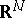,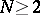, that depends non-linearly on the generating measure.

For example, in the study of properties of solutions of partial differential equations and of boundary properties of analytic functions, non-linear potentials of the following form turn out to be useful: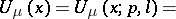(*)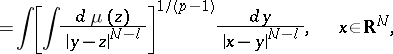whereis the distance betweenand,is a Radon measure with compact support, andandare real numbers,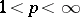,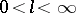.

Forthe non-linear potentials (*) turn into the linear Riesz potentials (cf. Riesz potential), and for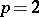and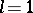into the classical Newton potential. The concepts of capacity and energy have been constructed, and analogues of certain basic theorems of potential theory have been proved for the non-linear potential (*) (see ).

How to Cite This Entry:
Non-linear potential. Encyclopedia of Mathematics. URL: http://encyclopediaofmath.org/index.php?title=Non-linear_potential&oldid=18320
This article was adapted from an original article by E.D. Solomentsev (originator), which appeared in Encyclopedia of Mathematics - ISBN 1402006098. See original article